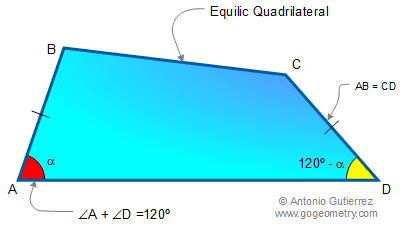Home > Problems >Equilic Quadrilateral > Previous, 1, 2, 3, 4, 5, Next

# Math: Geometry: Equilic Quadrilateral

## Definition

Equilic quadrilateral is a quadrilateral ABCD with one pair of opposite sides equal (AB = CD) and the sum of angles A and D equal to 120°.
Reference: Garfunkel, J. "The Equilic Quadrilateral." Pi Mu Epsilon J. 7, 317-329, 1981.Home | SearchGeometry | Problems | Equilic Quadrilateral | Post a comment | Email | by Antonio Gutierrez